# Get these flashcards, study & pass exams. For free! Even on iPhone/Android!

## IGCSE Physics Electricity and Magnetism (14 Cards)

What does Flemings left hand rule tell you about?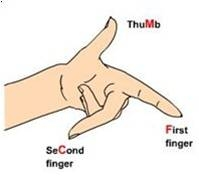It tells you about the motor effect. E.g which way a current carrying wire will move when inside a magnetic field.

on the picture:
"M" tells you which way the wire will move
"F" is the direction the field points
"C" is the direction the current flows (conventional current)
Describe the three pins on a transistor.
Transistors are composed of three parts – a base, a collector, and an emitter. The base is the gate controller device for the larger electrical supply. The collector is the larger electrical supply, and the emitter is the outlet for that supply.
Describe the three pins on a transistor.
Transistors are composed of three parts – a base, a collector, and an emitter. The base is the gate controller device for the larger electrical supply. The collector is the larger electrical supply, and the emitter is the outlet for that supply.
What does the word parallel mean in terms of electricity?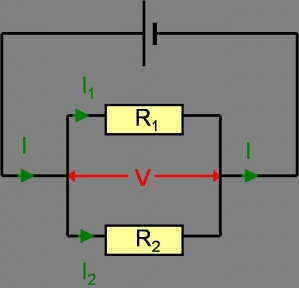How do resistances add up in parallel?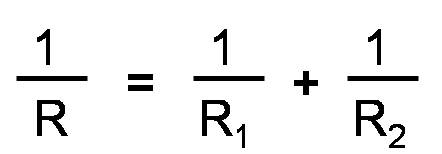What do resistors in series look like?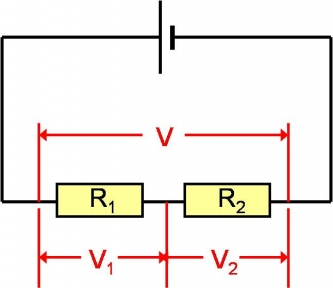What is the formula for electrical power?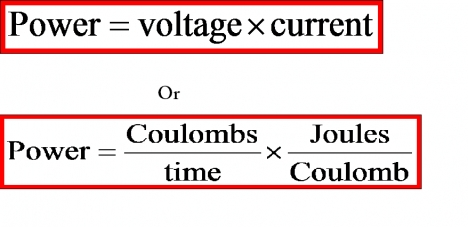How is current defined?
Number of coulombs of electrical charge passing a point every second.

I = Q/t

How is voltage defined?
Number of joules per Coulomb of charge

Voltage = joules/coulombs
Define E.M.F:
Electromotive force is the number of joules of chemical energy transferred to electrical  energy for each coulomb of charge that passes through a battery or cell.

CIE definition
What is Ohm's Law?
Voltage = current x Resistance
How do you remember the field around a current carrying wire?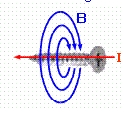Use the right hand screw rule.
The direction the screw is pointing is the direction the current flows, the direction you have to turn the screw is the direction the field is turning.
How do you remember the field around a current carrying coil of wire?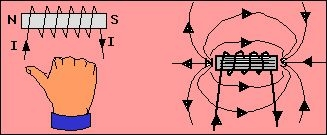The right hand rule tells you the polarity of a solenoid. The thumb points north and the fingers wrap around in the same direction as the conventional current.
What does fleming's right hand tell you?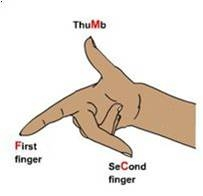The dynamo effect. The right hand rule can tell you the direction of induced current in cases where field direction and movement direction are known.

on the picture:
"M" tells you which way the wire will move
"F" is the direction the field points
"C" is the direction the current flows (conventional current)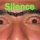Flashcard set info:
Author: Kamal
Main topic: Physics
School / Univ.: KNES
City: Kuwait
Published: 17.12.2009

Card tags:
All cards (14)
no tagsReport abuse